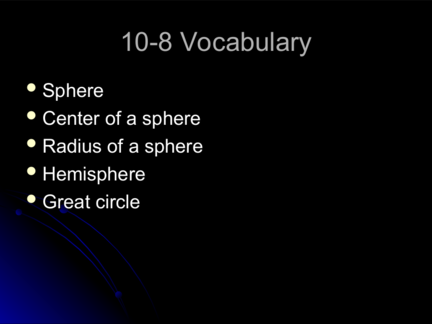# Volume of a SphereContributed by:This pdf contains:-
1. Sphere
2. Center of a sphere
3. The radius of a sphere
4. Hemisphere
5. Great circle
1. 10-8 Vocabulary
 Sphere
 Center of a sphere
 Hemisphere
 Great circle
2. 10-8 Spheres
Geometry
3. Sphere
 Sphere – is the locus of points in space that are
a given distance from a point. The point is
called the “center of the sphere”.
 Radius – a segment from the center to a point
on the sphere.
 Chord of a sphere – a segment whose
endpoints are on the sphere
 Diameter – a chord that contains the center
4. Volume of a Sphere
4 3
V  r
3
5. Example 1A
 Find
the volume of the sphere. Give your
d = 24 in
6. Example 1B
 Findthe diameter of a sphere with volume
36,000π cm³
7. More Definitions
 Ifa plane intersects a sphere and contains
the center, then the intersection is called a
great circle. Every great circle of a
sphere separates a sphere into two
congruent halves called hemispheres.
8. Example 2
 Findthe volume of the hemisphere with
9. Surface Area of a Sphere
2
S . A. 4r
10. Example 3A
Find the surface area of a sphere with a diameter of 76 cm.
What is the surface area of the sphere?
11. Example 3B
 Findthe volume of the sphere with a
surface area of 324π in².
12. Example 4
 Find
the surface area & volume of the